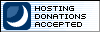# Vertex Details

## Overview

A vertex is a geometric point. A model triangle (or face) is defined by the three vertices that are connected to form the triangle. Triangles may share vertices. In general vertices are created when a tool is used to create triangles and deleted when all the triangles that use the vertex are deleted. However, it is possible to create a vertex independant of any triangle.

## Creating a Vertex

A vertex is created implicitly when triangles are created. Select any tool that creates a geometric shape (such as a cube, sphere, or cylinder) and it will create new vertices for the triangles that it creates. You may also create a vertex without any triangles attached by using the Create Vertex tool.

Note that a vertex that is created implicitly will be deleted implicitly when all the geometry that uses it is deleted. By contrast, a vertex that was created with the Create Vertex tool must be explicitly selected and deleted by the user. To find unused vertices, see the Select Free Vertices command.

## Other Vertex Details

If two triangles share a vertex and you want to force them to each have a separate vertex, you can use the Unweld Vertices command. If you want to combine two vertices into one, use the Weld Vertices command. command.

In order for weld to work, the vertices must be very close in 3D space. If the vertices are too far apart you can force them together by using the Snap Together command. You can also enable the Snap To Vertex feature to make it easier to line up vertices with each other.

Vertices can be attached to one or more bone joints to animate geometry in animation mode.